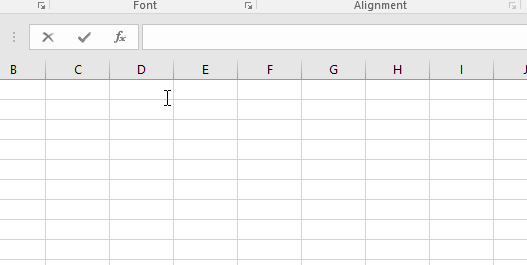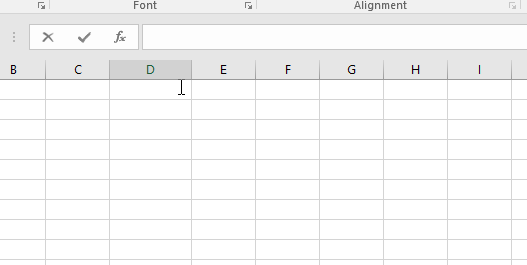# How to use the Excel PI function

The number ? is a mathematical constant. Originally defined as the ratio of a circle's circumference to its diameter, it now has various equivalent definitions and appears in many formulas in all areas of mathematics and physics. It is approximately equal to 3.14159.

Excel PI function returns the value of pi ? which is 3.14159
Syntax:

=PI()Let’s understand this function using an example.

As we know in mathematics

SIN(?/2)=1
So we will test this excel.
Use the formula

=SIN( PI() / 2 )

Here PI function returns the value of PI into the input of SIN function as a result we could get the value of SIN(?/2).Here we go, the PI function works great with other functions.

Hope you understood how to use the PI function in Excel. Explore more articles on Excel mathematical function here. Please feel free to state your query or feedback for the above article.

Related Articles:

How to use the Excel LOG10 function

How to use the Excel LN function

How to use the LOG function in Excel

How to use the Excel COS function

Popular Articles:

How to use the VLOOKUP Function in Excel

How to use the COUNTIF function in Excel 2016

Terms and Conditions of use

The applications/code on this site are distributed as is and without warranties or liability. In no event shall the owner of the copyrights, or the authors of the applications/code be liable for any loss of profit, any problems or any damage resulting from the use or evaluation of the applications/code.